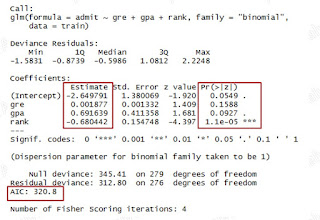## Pages

###R Tutorial 16.0

Vinod and I had struggled a lot to learn Logistic Regression in my time, but then we decided one thing that we would ensure no one struggles too much to learn about it. Hence we wrote a series of articles to explain it and covered the theory of Logistic model along with model building on SAS, let's now understand the same with R.

Series of previous Logistic Regression Modelling blogs at Ask Analytics.

### An Explicit coverage on ROC curve

____________________________________________________________________

Let's use the data available at site of Institute for Digital Research and Education for sake of simplicity.

# Let's prepare a dataset first

# We can see here that the data contains 4 columns : admit, gre, gpa and rank.
# Let's consider that the admit is dependent on gre score, gpa and rank. We will try to build a model on this data.

# Let's first do some EDA (exploratory data analysis) on the data and try to make some sense with the data. It might not make much sense here, but in real data it sure does.
# Infact, we first formulate few hypothesis such as:
# Higher the GRE score, more is chance of admission, higher the gpa, more is the chance of admission and with the EDA, we try to check our assumptions.

#Cross Tab
xtabs(~admit + rank, data = data_1)
xtabs(~admit + gre, data = data_1)
xtabs(~admit + gpa, data = data_1)

# Infact, based on the above cross tabs, we can convert the continous x variables into buckets, which segregate the 1 and 0 efficiently. We can otherwise use WOE and IV method to make the buckets of variables. Here we are not doing any bucketing.

# Lets now break the data into two parts : training data and validation data.
# We will train/build the model on the training data and then would check it on validation one. # We are breaking data in 70:30 ratio here.

dt = sort(sample(nrow(data_1), nrow(data_1)*.7))
train = data_1[dt,]
val= data_1[-dt,]

# Let's  now build the first logistic modelclick to enlarge

model_1 = glm(admit ~ gre + gpa + rank, data = train, family = "binomial")
summary(model_1)

# We can see in the result that gre is not coming as significant variable. Important stats that we need to look upon are highlighted.

#Both deviance and AIC are the measures of badness of the model, lesser these are, better the model is. But their stand alone values are not important, we need to check the values across models.

# The syntax of the logisitc regression is very simple
# model = glm( y ~ x1+x2+x3 ..., data = data_name, family = "binomial")

### Auto Selection of the model

# We can use either of backward, forward and step wise selection methods

model_2= step(model_1)   # Default selection method is backward
summary(model_2)
model_2= step(model_1, direction="forward")
summary(model_2)
model_2= step(model_1, direction="both")
summary(model_2)

model_2= step(model_1, direction="both")
summary(model_2)

# You can notice that gre variables has been removed from the model as it was not having sufficient significance (p value) to be in the model.

# Here AIC value has increased, but Null deviance has decreased. Generally, both the values change in the same direction and decrease as you improve the model. But sometimes, we need to compromise, as it is more important to drop the insignificant variables.

Let's assume we stop at model_2, we can now check the odd ratio estimates.

exp(coef(model_2))

#Lets use the model to calculate the probability of admission both for training and validation data

train\$probability = predict(model_2, type="response") # predicted values
val\$probability = predict(model_2, newdata = val, type="response")

### Model diagnostic check up

H-L Test

library(ResourceSelection)

# The null hypothesis of the H-L test is " Observed and expected events are same", P value of the H-L test should be more than 0.05 in order to accept the null hypothesis and hence the model.

install.packages("pROC")
library(pROC)
plot(g6)

# The are under the ROC curve signifies the health of the model. More the area, better the model is.

# Also it is used to decide the probabilty cut-off value.

Confusion Matrix
install.packages("caret")
install.packages("e1071")
library(caret)
library(e1071)
train\$y_hat= ifelse(train\$probability>0.5,1,0)

# We can build the confusion matrix at given cut-off probability level, here 0.5.

# We are keeping the article restricted to R coding and its result part as the most of the explanation part is already covered in the blog enlisted in the starting.

and finally we are done with logistic regression in R, please let us know if you want to add anything else in the article.

Enjoy reading our other articles and stay tuned with us.

Kindly do provide your feedback in the 'Comments' Section and share as much as possible.